CLOSE

Recommanded Free YOUTUBE Lecture: <% selectedImage %>

## Contents

### 선형회귀

모두를 위한 머신러닝/딥러닝에서 텐서플로우(TensorFlow)부분을 보려고 하니 선형회귀(Linear Regression)라는 녀석이 튀어나왔다. 이 녀석 부터 살펴보기로 했다.

선형회귀는 종속 변수 y와 한 개 이상의 독립 변수 X와의 선형 관계를 모델링하는 회귀분석 기법이다. 한 개의 설명 변수에 기반한 경우에는 단순 선형회귀, 둘 이상의 설명 변수에 기반한 경우에는 다중 선형 회귀라 한다. 회귀분석이란 한 변수에 대한 다른 변수의 변화를 예측할 때, 그 관계를 함수관계(Y=F(Xs))로 나타내는 분석을 말한다.

회귀분석은 점들이 퍼져있는 형태에서 패턴을 찾아내고, 이 패턴을 활용해서 무엇인가를 예측하는 분석 기버비다. 새로운 표본을 뽑았을 때 평균으로 돌아가려는(회귀) 특징이 있기 때문에 붙은 이름이다.

### 단순 선형회귀

첫번째 변수 X 로 부터 두번째 변수 Y의 점수를 예측을 하려 한다. 여기에서 예측하려는 변수 Ycriterion variable 혹은 독립변수(dependent variable)라고 부른다. 그리고 예상을 하기 위해서 토대로 삼는 X 항의 변수를 predictor variable이라고 한다. 단지 하나의 predictor variable만을 가지는 것을 단순 선형 회귀라고 한다.

아래는 단순 선형회귀를 위해서 사용한 예제 데이터다.
 X Y 1.00 1.00 2.00 2.00 3.00 1.30 4.00 3.75 5.00 2.25
이것을 그래프로 나타내보자.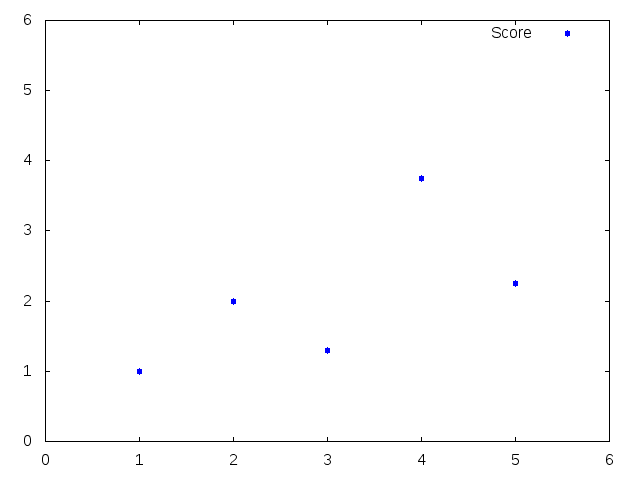선형회귀는 각 포인트들을 통과하는 가장 잘 정돈된 직선을 찾는게 목적이다. 이 직선을 회귀선이라고 한다. 아래 그림은 위에 있는 데이터를 기반으로 만들어진 회귀선을 보여주고 있다. 이 그림은 회귀직선과 이 직선에서의 예측 오류를 나타내는 점수정보를 포함하고 있다. 회귀선에서 가까울 수록 예측 오류가 작음을 나타낸다. X(1)의 예측 오차는 -0.210으로 가장 작고 X(4)의 예측 오차는 1.265로 가장 크다.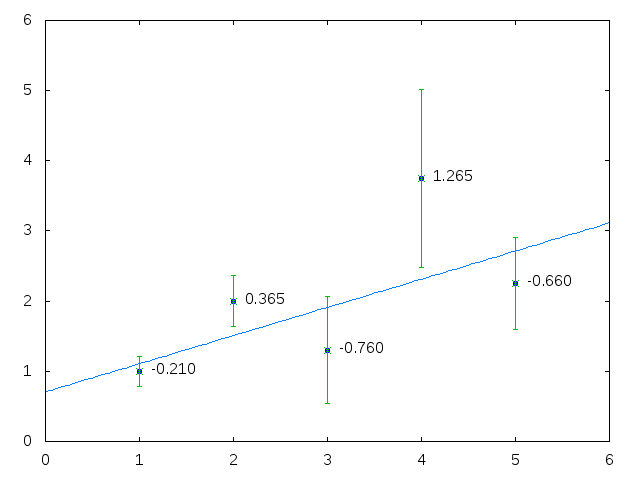위 그래프를 표로 정리를 했다. Y1 은 예측 값이고, Y-Y1는 예측 오류다.
 X Y Y1 Y-Y1 (Y-Y1)^2 1.00 1.00 1.210 -0.210 0.044 2.00 2.00 1.635 0.365 0.133 3.00 1.30 2.060 -0.760 0.578 4.00 3.75 2.485 1.265 1.600 5.00 2.25 2.910 -0.660 0.436

#### Hypothesis

위 표에서회귀직선을 나타내는 회귀방정식은 로 나타낼 수 있다. 이 일차방정식을 (가설)Hypothesis이라고 하며 H(x) 혹은 h(x)로 표현한다. 에서 Wx+b는 x에 대한 1차 방정식으로 직선을 표현한다는 것을 알고 있다. W는 기울기이고 b는 절편이다. W와 b가 반복되는 과정에서 계속 바뀌고, 최종 값을 사용해서 예측에 사용 할 수 있게 된다. 위 데이터는 따르고 있다. 예를 들어서 X = 1 이라면 H(1) = (0.425 * 1) + 0.785 = 1.21 이다. X = 2 라면 H(2) = (0.425 * 2) + 0.785 = 1.635 가된다.

b와 Y는 통계 소프트웨어를 이용해서 간단하게 구 할 수 있다. python scipy를 이용해서 구해보자.
from scipy import stats, polyval
x= [1,2,3,4,5]
y =[1, 2, 1.30, 3.75, 2.25]

slope, intercept, r, p, std = stats.linregress(x,y)
ry = polyval([slope, intercept], x)

print(slope, intercept, r, p, std)
print(ry)
실행 결과 0.425와 0.784를 확인 할 수 있다.
# python regress.py
(0.42500000000000004, 0.78499999999999992, 0.62683274897895758, 0.2577773028538885, 0.30499999999999999)
[ 1.21   1.635  2.06   2.485  2.91 ]

여기에서는 그냥 python을 이용해서 계산을 했는데, 이 계산 방법을 수학적으로 찾아보자.

#### Cost Function

우리가 세운 가설은 이다. 예측값에서 실제 값을 빼주면 된다. 하지만 단순히 빼거나 더 할 경우 음수가 나올 수가 있어서 계산이 복잡해 질 수 있으므로 제곱을 해서 양수로 만들어 준다. 제곱을 하는 이유는 아래와 같다.
1. 뺄셈을 하면 데이터의 위치에 따라 음수와 양수가 섞인다. 계산하기가 피곤해진다.
2. 절대 값을 취할 수도 있지만 제곰을 하는 방법도 있다. (음수 X 음수), (양수 X 양수) 모드 양수가 나오기 때문이다.
3. 제곱을 할 경우 멀리 있는 값이 더 큰 값이 나온다. 따라서 멀리있는 데이터에 벌점(Penalty)를 줄 수 있다.
4. 다음 n으로나눈다. 데이터가 많아지면 데이터가 너무 커질 수 있는데, 값을 평균으로 만들면 계산하기가 편해진다.
우리는 원본 데이터로 부터 아래와 같은 식을 만들 수 있다.

이 식을 일반화 하면 이 된다. 이렇게 해서 W,b에 대한 코스트 함수 가 만들어진다. 여기에서 거리를 가장 작게 만들어주는 W와 b를 구하면 된다. 이 과정이 선형회귀에서의 학습이다.

머신러닝은 데이터를 학습 하면서, 최적화된 값을 찾는 일련의 과정이다. 최적화된 값을 찾기 위해서는 오차를 계속 줄여가기 위한 어떤 방법을 개발해야 한다. 이것은 한치앞이 안보이는 울창한 밀림에서 계곡으로 가야 한다고 가정해 보자. 앞이 보이지 않기 때문에 계곡이 어디있는지 알 수 없지만 현재 위치에서 경사가 아래로 가파른쪽으로 내려가다 보면 결국 계곡에 다다르게 될 것이다. 이렇게 극소점을 찾기 위해 이동해 가는 방법을 경사하강법(Gradient descent) 라고 부른다. 이걸 수학적으로 풀어보자.우리는 검은색 공을 그래프의 밑바닥으로 옮겨야 한다. 공이 밑으로 가고 있는 지는 공이 위치한 지점에서의 기울기(Gradient)가 이전 기울기 보다 낮아지는 지를 검사하는 것으로 판단 할 수 있다. 이렇게 기울기가 줄어드는 방향으로 움직이다가 0이 되는 지점을 찾으면 여기가 그래프의 밑바닥이 된다. 아래 그림을 보자.경사하강법을 이용해서 어떻게 원하는 곳을 찾아가는지를 묘사하고 있다. 이제 경사하강법을 알고리즘으로 나타내보자. 변화율이므로 미분 방정식으로 표현된다.

는 learning rate로 얼마나 빠르게 내려올지 결정하기 위해서 사용한다. 은 편미분(partial derivative)하라는 의미다. 이 과정을 수렴할 때까지 반복 진행하면 된다. 미분이라고 해서 겁먹을 필요는 없다. Tensorflow 라이브러리에서 제공하는 함수를 호출하기만 하면 된다.
a = tf.Variable(0.1)
train = optimizer.minimize(cost)

### 예제

SAT and College GPA미국 105대학 및 고등학교의 GPA(Grade Point Average - 평균학점)과 컴퓨터와 수학에 대한 SAT 점수를 포함하고 있다. 이 정보를 이용해서 고등학교에서의 GPA로 대학에서의 GPA를 예측해보자.

#### GNU Plot

TensorFlow를 이용하기 전에 GNUPlot로 분석해 보자. 많지 않은 데이터에 대한 간단한 선형회귀 정도는 GNUPlot를 이용해서 분석 할 수 있다. 위 XLS 버전의로 테스트 했다. 아래는 gnuplot 스크립트 파일이다.
# cat sat.dem
set xlabel "High School GPA"
set ylabel "University GPA"

# 선형회귀 분석 모델
f(x) = m*x + c

# 분석 모델에 사용할 데이터를 추출한다.
fit m*x + c "sat.dat" using 1:5 via m, c

# plotting 한다.
plot f(x) title 'Line Fit'
plot "sat.dat" using 1:5 title 'GPA'  with points, f(x) title 'Model Fit'
pause -1
gnuplot로 실행하면, f(x) = m*x+c모델링 결과가 표준출력되는 걸 확인 할 수 있다.
# gnuplot sat.dem
......
Final set of parameters            Asymptotic Standard Error
=======================            ==========================

m               = 0.67483          +/- 0.05342      (7.916%)
c               = 1.09682          +/- 0.1666       (15.19%)
......
0.67483과 1.09682를 얻었다. 그래프를 보자.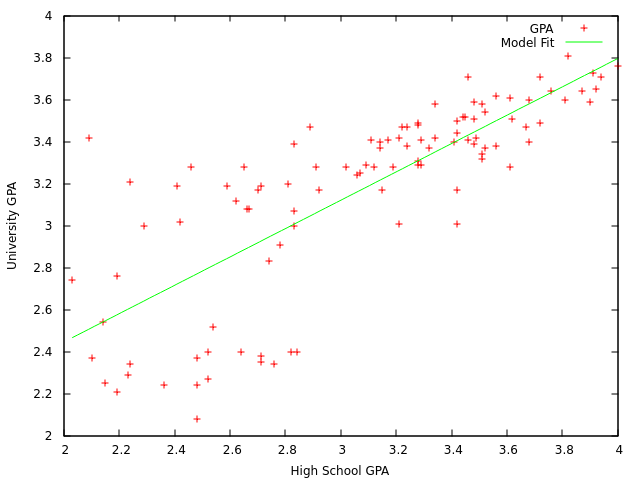이제 고등학교의 GPA가 3일 경우 대학교의 예상 GPA는 (0.67483 * 3) + 1.09682 == 3.12131임을 예측 할 수 있다.

#### python scipy

python 버전이다. 설명은 주석으로 대신한다.대신한다.대신한다.대신한다.
#!/usr/bin/python
from scipy import stats, polyval
import numpy as np
import matplotlib.pyplot as plt

// 파일을 줄 단위로 읽은 후 공백을 기준으로 split 한 후,
// 고등학교와 대학교의 GPA를 배열에 넣는다.
f = open("sat.txt", "r")
num = 0
h_GPA = []
u_GPA = []
while True:
if not line: break
items = line.split()
h_GPA.append(float(items))
u_GPA.append(float(items))
f.close()

slope, intercept, r, p, std = stats.linregress(h_GPA,u_GPA)
ry = polyval([slope, intercept], h_GPA)

print(slope, intercept, r, p, std)

// 그래프를 그린다.
plt.plot(h_GPA, u_GPA, '.')
plt.plot(h_GPA, np.array(h_GPA) * slope + intercept )
plt.show()
실행 결과를 보자.
# ./sat.py
(0.67482990344776062, 1.0968232817933754, 0.77956312054891574, 1.1756126028764049e-22, 0.053422381816411617)
아래는 그래프다.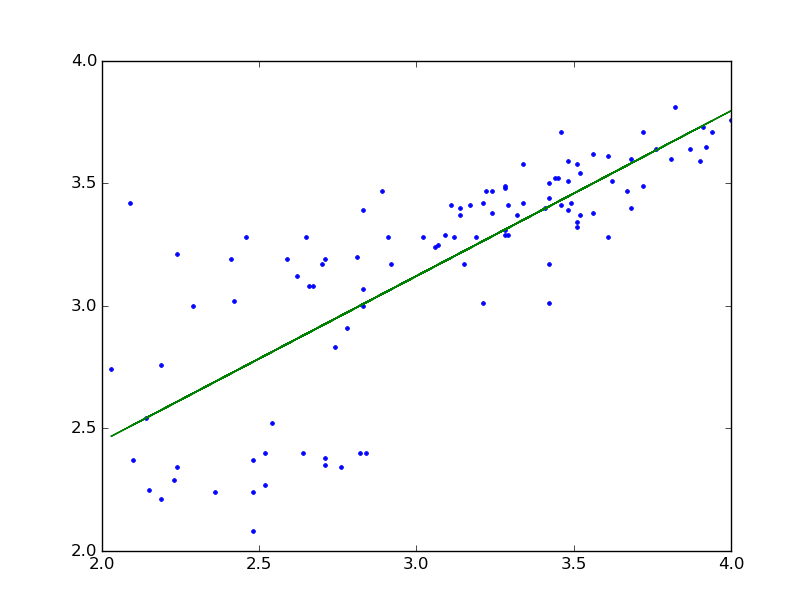### TensorFlow

이제 TensorFlow를 이용해서 테스트해보자.

설치는 TensorFlow 시작문서를 참고하자.이제 TensorFlow를 이용해서 GPA 문제를 풀어보자. 아래는 앞서 테스트한 python 코드의 일부분이다.
plt.plot(h_GPA, u_GPA, '.')
plt.plot(h_GPA, np.array(h_GPA) * slope + intercept )
TensorFlow를 이용해서 slopeintercept를 구하면 된다. 우리는 이미 slope가 0.67483 이고 intercept가 1.09682 라는 것을 알고 있다. 하지만 TensorFlow는 이 값을 모르고 있으므로 직접 찾아야 한다.

TensorFlow는 데이터셋의 값들을 반복 적용하는 사이클을 거치면서 더 정확한 결과를 얻을 수 있도록 slope 와 intercept 를 수정하는 식으로 작동한다. 사이클이 돌 때마다 모델이 개선되고 있는지를 확인 하기 위해서 코스트 함수(Cost Function)를 정의해야 한다.

우리는 선형회구에서의 코스트 함수가 평균 제곱 편차(mean square error -MSE)라는 걸 알고 있다. 즉

이 된다.

이제 TensorFlow로 코드를 만들면 된다.
from scipy import stats, polyval
import numpy as np
import matplotlib.pyplot as plt
import tensorflow as tf

f = open("sat.txt", "r")
num = 0
vectors_set=[]
while True:
if not line: break
items = line.split()
vectors_set.append([float(items), float(items)])
f.close()

x_data = [v for v in vectors_set]
y_data = [v for v in vectors_set]

plt.plot(x_data, y_data, '.')
plt.legend()
plt.show()

W = tf.Variable(tf.random_uniform(, -1.0, 0.0))
b = tf.Variable(tf.zeros())
y = W * x_data + b

loss = tf.reduce_mean(tf.square(y - y_data))
train = optimizer.minimize(loss)

init = tf.initialize_all_variables()

sess = tf.Session()
sess.run(init)

for step in xrange(8):
sess.run(train)
print(step, sess.run(W), sess.run(b))
print(step, sess.run(loss))

#Graphic display
plt.plot(x_data, y_data, '.')
plt.plot(x_data, sess.run(W) * x_data + sess.run(b))

plt.show()

알고리즘을 반복(데이터를 트레이닝)하면서 적합한 모델을 만드는 것을 확인 할 수 있다.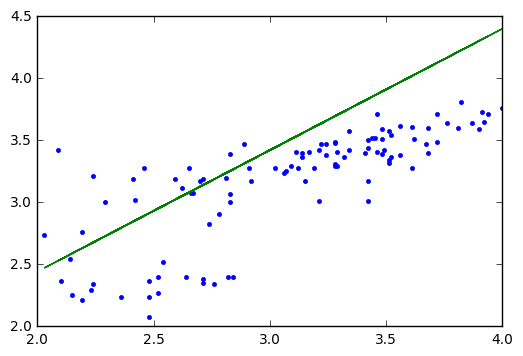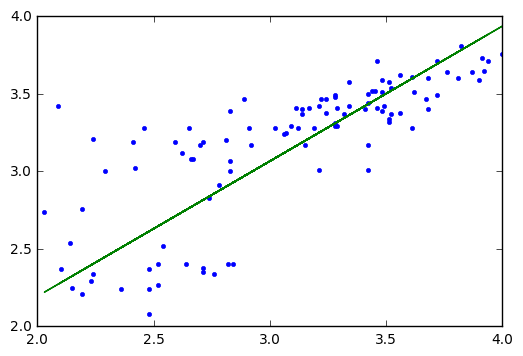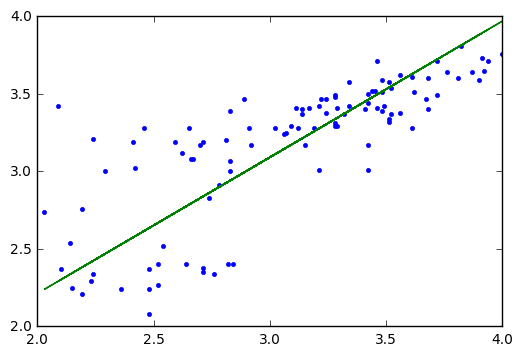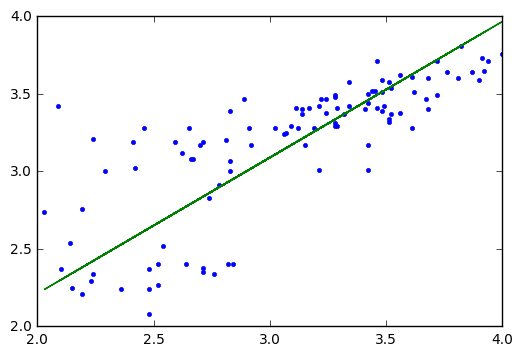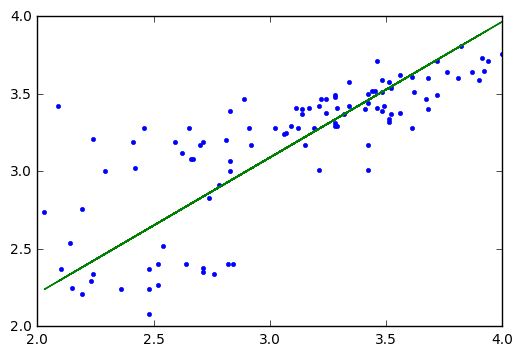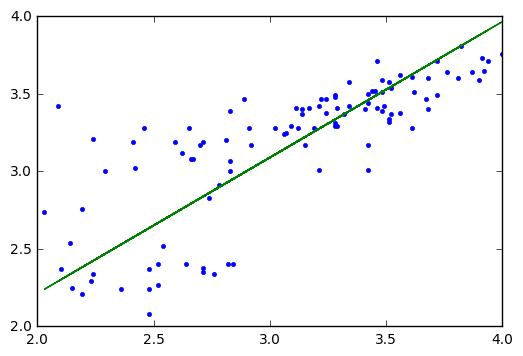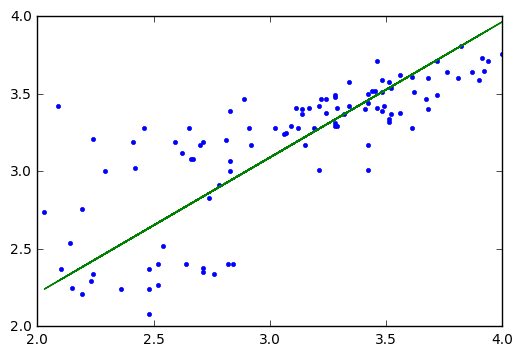### 선형회귀분석의 특징들

• 선형회귀는 데이터가 직선을 따르는 경향이 있다고 가정한다. 이러한 경향을 따르지 않는 데이터의 경우 정확도가 떨어질 수 있다.
• 간단하고 학습시간이 빠르다.
• 정규분포 타입의 데이터에 적용하기 좋다.